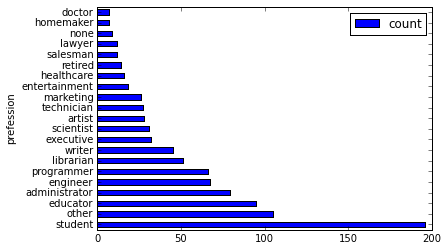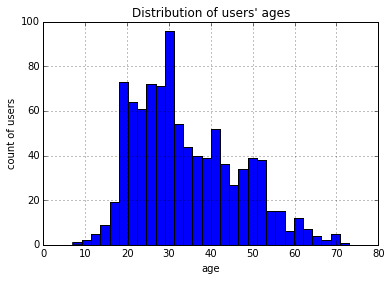## 获取PyODPS项目空间

• 获取项目空间：使用MaxCompute入口对象的`get_project()`方法获取项目空间。DataWorks的PyODPS节点中，将会包含一个全局变量`odps`或者`o`，即为MaxCompute入口。您不需要手动定义MaxCompute入口。
``````project = o.get_project('project_name')  # 指定项目空间时，获取特定项目。
project = o.get_project()              # 不指定项目空间时，获取当前项目。``````

该方法需要提供project_name，即项目空间名称。

• 验证项目空间是否存在：使用`exist_project()`方法检验某个项目空间是否存在。

## 基本表操作

• 通过调用入口对象的`list_tables()`方法可以列出项目空间下的所有表。
``````# 处理每张表。
for table in odps.list_tables():``````
• 通过调用入口对象的`exist_table()`方法判断表是否存在；通过调用`get_table()`方法获取表。
``````t = odps.get_table('dual')
t.schema
odps.Schema {
c_int_a                 bigint
c_int_b                 bigint
c_double_a              double
c_double_b              double
c_string_a              string
c_string_b              string
c_bool_a                boolean
c_bool_b                boolean
c_datetime_a            datetime
c_datetime_b            datetime
}
t.lifecycle
-1
print(t.creation_time)
2014-05-15 14:58:43
t.is_virtual_view
False
t.size
1408
t.schema.columns
[<column c_int_a, type bigint>,
<column c_int_b, type bigint>,
<column c_double_a, type double>,
<column c_double_b, type double>,
<column c_string_a, type string>,
<column c_string_b, type string>,
<column c_bool_a, type boolean>,
<column c_bool_b, type boolean>,
<column c_datetime_a, type datetime>,
<column c_datetime_b, type datetime>]            ``````

## 创建表的Schema

• 通过表的列以及可选的分区进行初始化。
``````from odps.models import Schema, Column, Partition
columns = [Column(name='num', type='bigint', comment='the column'),
Column(name='num2', type='double', comment='the column2')]
partitions = [Partition(name='pt', type='string', comment='the partition')]
schema = Schema(columns=columns, partitions=partitions)
schema.columns
[<column num, type bigint>,
<column num2, type double>,
<partition pt, type string>]
schema.partitions
[<partition pt, type string>]
schema.names  # 获取非分区字段的字段名。
['num', 'num2']
schema.types  # 获取非分区字段的字段类型。
[bigint, double]``````
• 使用`Schema.from_lists()`方法。该方法更容易调用，但无法直接设置列和分区的注释。
``````schema = Schema.from_lists(['num', 'num2'], ['bigint', 'double'], ['pt'], ['string'])
schema.columns
[<column num, type bigint>,
<column num2, type double>,
<partition pt, type string>]``````

## 创建表

• 使用表Schema创建表，方法如下。
``````table = o.create_table('my_new_table', schema)
table = o.create_table('my_new_table', schema, if_not_exists=True)  # 只有不存在表时，才创建表。
table = o.create_table('my_new_table', schema, lifecycle=7)  # 设置生命周期。``````
• 采用字段名及字段类型字符串创建表，方法如下。
``````# 创建非分区表。
table = o.create_table('my_new_table', 'num bigint, num2 double', if_not_exists=True)
# 创建分区表可传入（表字段列表，分区字段列表）。
table = o.create_table('my_new_table', ('num bigint, num2 double', 'pt string'), if_not_exists=True)``````
未打开新数据类型开关时，创建表的数据类型只允许为BIGINT、DOUBLE、DECIMAL、STRING、DATETIME、BOOLEAN、MAP和ARRAY类型。如果您需要支持TINYINT和STRUCT等新数据类型，可以打开`options.sql.use_odps2_extension = True`开关，示例如下。
``````from odps import options
options.sql.use_odps2_extension = True
table = o.create_table('my_new_table', 'cat smallint, content struct<title:varchar(100), body:string>')``````

## 删除表

``````o.delete_table('my_table_name', if_exists=True)  # 只有表存在时，才删除表。
t.drop()  # Table对象存在时，直接调用Drop方法删除。``````

## 表分区

• 判断是否为分区表。
``````if table.schema.partitions:
print('Table %s is partitioned.' % table.name)``````
• 遍历表全部分区。
``````for partition in table.partitions:
print(partition.name)
for partition in table.iterate_partitions(spec='pt=test'):
# 遍历二级分区。``````
• 判断分区是否存在。
``table.exist_partition('pt=test,sub=2015')``
• 获取分区。
``````partition = table.get_partition('pt=test')
print(partition.creation_time)
2015-11-18 22:22:27
partition.size
0``````
• 创建分区。
``t.create_partition('pt=test', if_not_exists=True)  # 指定if_not_exists参数，分区不存在时才创建分区。``
• 删除分区。
``````t.delete_partition('pt=test', if_exists=True)  # 指定if_exists参数，分区存在时才删除分区。
partition.drop()  # 分区对象存在时，直接对分区对象调用Drop方法删除。``````

## PyODPS SQL

PyODPS支持MaxCompute SQL查询，并可以读取执行的结果。
• 执行SQL。
入口对象的`execute_sql('statement')``run_sql('statement')`方法可以执行SQL语句，返回值请参见任务实例
``````odps.execute_sql('select * from dual')  #  同步的方式执行，会阻塞直到SQL执行完成。
instance = odps.run_sql('select * from dual')  # 异步的方式执行。
instance.wait_for_success()  # 阻塞直到完成。``````
• 读取SQL执行结果。
运行SQL的Instance能够直接执行`open_reader`操作读取SQL执行结果。读取时会出现以下两种情况：
• SQL返回了结构化的数据。
``````with o.execute_sql('select * from dual').open_reader() as reader:
# 处理每一个record。``````
• SQL可能执行了`desc`命令，这时可以通过`reader.raw`取到原始的SQL执行结果。
``````with o.execute_sql('desc dual').open_reader() as reader:

## PyODPS资源

MaxCompute中的资源常用在UDF和MapReduce中。基本操作如下：
• `list_resources()`：列出该项目空间下的所有资源。
• `exist_resource()`：判断资源是否存在。
• `delete_resource()`：删除资源。您也可以通过Resource对象调用`drop`方法实现。
• `create_resource()`：创建资源。
• `open_resource()`：读取资源。

• 文件资源
文件资源包括基础的`FILE``PY``JAR``ARCHIVE`
说明 在DataWorks中，PY格式的文件资源请以FILE形式上传，详情请参见Python UDF文档
文件资源常见操作如下：
• 创建文件资源

您可以通过给定资源名、文件类型和一个file-like的对象（或字符串对象）调用`create_resource()`来创建。

``````resource = o.create_resource('test_file_resource', 'file', file_obj=open('/to/path/file'))  # 使用file-like的对象创建文件资源。
resource = o.create_resource('test_py_resource', 'py', file_obj='import this')  # 使用字符串创建文件资源。``````
• 读取和修改文件资源
打开一个资源有如下两种方法：
• 对文件资源调用`open`方法。
• 在MaxCompute入口调用`open_resource()`方法。
打开后的对象是file-like的对象。类似于Python内置的`open()`方法，文件资源也支持打开的模式，示例如下。
``````with resource.open('r') as fp:  # 以读模式打开资源。
fp.seek(0)  # 回到资源开头。
fp.write('Hello World')  # 报错，读模式下无法写资源。

with o.open_resource('test_file_resource', mode='r+') as fp:  # 读写模式打开资源。
fp.tell()  # 定位当前位置。
fp.seek(10)
fp.truncate()  # 截断后面的内容。
fp.writelines(['Hello\n', 'World\n'])  # 写入多行。
fp.write('Hello World')
fp.flush()  # 手动调用会将更新提交到ODPS。``````

所有支持的打开类型包括：

• `r`：读模式，只能打开不能写。
• `w`：写模式，只能写入而不能读文件，注意用写模式打开，文件内容会先被清空。
• `a`：追加模式，只能写入内容到文件末尾。
• `r+`：读写模式，可以任意读写内容。
• `w+`：类似于`r+`，但会先清空文件内容。
• `a+`：类似于`r+`，但写入时只能写入文件末尾。
同时，PyODPS中文件资源支持以二进制模式打开，例如一些压缩文件需要以这种模式打开。
• `rb`：指以二进制读模式打开文件。
• `r+b`：指以二进制读写模式打开。
• 表资源
• 创建表资源
``o.create_resource('test_table_resource', 'table', table_name='my_table', partition='pt=test')``
• 更新表资源
``````table_resource = o.get_resource('test_table_resource')
table_resource.update(partition='pt=test2', project_name='my_project2')``````
• 获取表及分区
``````table_resource = o.get_resource('test_table_resource')
table = table_resource.table
print(table.name)
partition = table_resource.partition
print(partition.spec)``````
• 读写内容
``````table_resource = o.get_resource('test_table_resource')
with table_resource.open_writer() as writer:
writer.write([0, 'aaaa'])
writer.write([1, 'bbbbb'])
print(rec)``````

## DataFrame

PyODPS提供了DataFrame API，它提供了类似Pandas的接口，但是能充分利用MaxCompute的计算能力。完整的DataFrame文档请参见DataFrame

1. 首先创建MaxCompute的入口对象。
``````#创建MaxCompute入口对象。
o = ODPS('**your-access-id**', '**your-secret-access-key**',project='**your-project**', endpoint='**your-end-point**'))``````
2. 传入Table对象，创建DataFrame对象users。
``````from odps.df import DataFrame
users = DataFrame(o.get_table('pyodps_ml_100k_users'))``````
3. 对DataFrame对象可以执行如下操作：
• 通过dtypes属性可以查看DataFrame的字段和类型，如下所示。
``users.dtypes``
``users.head(10)``
返回结果如下。
- user_id age sex occupation zip_code
0 1 24 M technician 85711
1 2 53 F other 94043
2 3 23 M writer 32067
3 4 24 M technician 43537
4 5 33 F other 15213
5 6 42 M executive 98101
6 7 57 M administrator 91344
7 8 36 M administrator 05201
8 9 29 M student 01002
9 10 53 M lawyer 90703
• 对字段进行筛选。
• 筛选部分字段。
``users[['user_id', 'age']].head(5)``
返回结果如下。
- user_id age
0 1 24
1 2 53
2 3 23
3 4 24
4 5 33
• 排除个别字段，如下所示。
``>>> users.exclude('zip_code', 'age').head(5)``
返回结果如下。
- user_id sex occupation
0 1 M technician
1 2 F other
2 3 M writer
3 4 M technician
4 5 F other
• 排除掉一些字段的同时，通过计算得到一些新的列。例如，将sexM设置为True，否则设置为False，并将此列取名为sex_bool。如下所示。
``>>> users.select(users.exclude('zip_code', 'sex'), sex_bool=users.sex == 'M').head(5)``
返回结果如下。
- user_id age occupation sex_bool
0 1 24 technician True
1 2 53 other False
2 3 23 writer True
3 4 24 technician True
4 5 33 other False
• 查询年龄在20~25岁之间的人数，如下所示。
``````>>> users.age.between(20, 25).count().rename('count')
943``````
• 查询男女用户的数量。
``>>> users.groupby(users.sex).count()``
返回结果如下。
- sex count
0 F 273
1 M 670
• 将用户按职业划分，从高到底，获取人数最多的前10个职业。
``````>>> df = users.groupby('occupation').agg(count=users['occupation'].count())
>>> df.sort(df['count'], ascending=False)[:10]``````
返回结果如下。
- occupation count
0 student 196
1 other 105
2 educator 95
4 engineer 67
5 programmer 66
6 librarian 51
7 writer 45
8 executive 32
9 scientist 31
DataFrame API提供了value_counts方法来快速达到同样的目的。
``>>> users.occupation.value_counts()[:10]``
返回结果如下。
- occupation count
0 student 196
1 other 105
2 educator 95
4 engineer 67
5 programmer 66
6 librarian 51
7 writer 45
8 executive 32
9 scientist 31
• 使用更直观的图来查看这份数据。
`` %matplotlib inline``
• 使用横向的柱状图来可视化。
``users['occupation'].value_counts().plot(kind='barh', x='occupation', ylabel='prefession')``• 使用直方图来可视化。将年龄分成30组，查看各年龄分布的直方图，如下所示。
``>>> users.age.hist(bins=30, title="Distribution of users' ages", xlabel='age', ylabel='count of users')``• 使用JOIN将三张表进行联合后，保存成一张新的表。
``````movies = DataFrame(o.get_table('pyodps_ml_100k_movies'))
ratings = DataFrame(o.get_table('pyodps_ml_100k_ratings'))
o.delete_table('pyodps_ml_100k_lens', if_exists=True)
lens = movies.join(ratings).join(users).persist('pyodps_ml_100k_lens')
lens.dtypes``````
结果如下。
``````odps.Schema {
movie_id                            int64
title                               string
release_date                        string
video_release_date                  string
imdb_url                            string
user_id                             int64
rating                              int64
unix_timestamp                      int64
age                                 int64
sex                                 string
occupation                          string
zip_code                            string
}``````
• 把0~80岁的年龄，分成8个年龄段。
``````labels = ['0-9', '10-19', '20-29', '30-39', '40-49', '50-59', '60-69', '70-79']
cut_lens = lens[lens, lens.age.cut(range(0, 81, 10), right=False, labels=labels).rename('年龄分组')]``````
• 取分组和年龄唯一的前10条数据来进行查看。
``>>> cut_lens['年龄分组', 'age'].distinct()[:10]``
结果如下。
- 年龄分组 age
0 0-9 7
1 10-19 10
2 10-19 11
3 10-19 13
4 10-19 14
5 10-19 15
6 10-19 16
7 10-19 17
8 10-19 18
9 10-19 19
• 对各个年龄分组下，用户的评分总数和评分均值进行查看，如下所示。
``cut_lens.groupby('年龄分组').agg(cut_lens.rating.count().rename('评分总数'), cut_lens.rating.mean().rename('评分均值'))``
结果如下。
- 年龄分组 评分均值 评分总数
0 0-9 3.767442 43
1 10-19 3.486126 8181
2 20-29 3.467333 39535
3 30-39 3.554444 25696
4 40-49 3.591772 15021
5 50-59 3.635800 8704
6 60-69 3.648875 2623
7 70-79 3.649746 197

## Configuration

PyODPS提供了一系列的配置选项，可通过`odps.options`命令获得。可配置的MaxCompute选项，如下所示。
• 通用配置
选项 说明 默认值
end_point MaxCompute Endpoint None
default_project 默认项目空间 None
log_view_host Logview主机名 None
log_view_hours Logview保持时间（小时） 24
local_timezone 使用的时区。True表示本地时间，False表示UTC，也可用pytz时区 1
lifecycle 所有表生命周期 None
temp_lifecycle 临时表生命周期 1
biz_id 用户ID None
verbose 是否打印日志 False
verbose_log 日志接收器 None
chunk_size 写入缓冲区大小 1496
retry_times 请求重试次数 4
pool_connections 缓存在连接池的连接数 10
pool_maxsize 连接池最大容量 10
connect_timeout 连接超时 5
completion_size 对象补全列举条数限制 10
notebook_repr_widget 使用交互式图表 True
sql.settings MaxCompute SQL运行全局hints None
sql.use_odps2_extension 启用MaxCompute 2.0语言扩展 False
• 数据上传或下载配置
选项 说明 默认值
tunnel.endpoint Tunnel Endpoint None
tunnel.use_instance_tunnel 使用Instance Tunnel获取执行结果 True
tunnel.limited_instance_tunnel 限制Instance Tunnel获取结果的条数 True
tunnel.string_as_binary 在STRING类型中使用Bytes而非Unicode False
• DataFrame配置
选项 说明 默认值
interactive 是否在交互式环境 根据检测值
df.analyze 是否启用非MaxCompute内置函数 True
df.optimize 是否开启DataFrame全部优化 True
df.optimizes.pp 是否开启DataFrame谓词下推优化 True
df.optimizes.cp 是否开启DataFrame列剪裁优化 True
df.optimizes.tunnel 是否开启DataFrame使用Tunnel优化执行 True
df.quote MaxCompute SQL后端是否用``来标记字段和表名 True
df.libraries DataFrame运行使用的第三方库（资源名） None
• PyODPS ML配置
选项 说明 默认值
ml.xflow_project 默认Xflow工程名 algo_public
ml.use_model_transfer 是否使用ModelTransfer获取模型PMML True
ml.model_volume 在使用ModelTransfer时使用的Volume名称 pyodps_volume